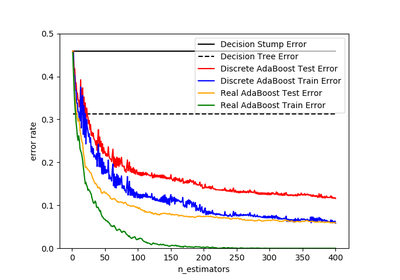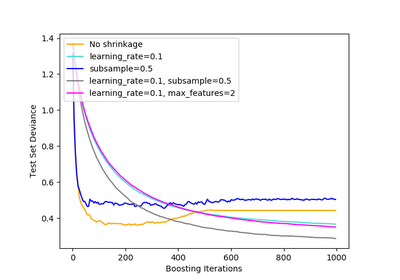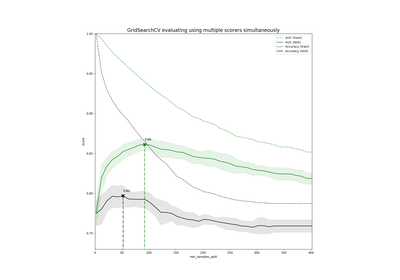# `sklearn.datasets`.make_hastie_10_2¶

`sklearn.datasets.``make_hastie_10_2`(n_samples=12000, random_state=None)[source]

Generates data for binary classification used in Hastie et al. 2009, Example 10.2.

The ten features are standard independent Gaussian and the target `y` is defined by:

```y[i] = 1 if np.sum(X[i] ** 2) > 9.34 else -1
```

Read more in the User Guide.

Parameters: n_samples : int, optional (default=12000) The number of samples. random_state : int, RandomState instance or None, optional (default=None) If int, random_state is the seed used by the random number generator; If RandomState instance, random_state is the random number generator; If None, the random number generator is the RandomState instance used by np.random. X : array of shape [n_samples, 10] The input samples. y : array of shape [n_samples] The output values.

`make_gaussian_quantiles`
a generalization of this dataset approach

References

 [R147] T. Hastie, R. Tibshirani and J. Friedman, “Elements of Statistical Learning Ed. 2”, Springer, 2009.

## Examples using `sklearn.datasets.make_hastie_10_2`¶